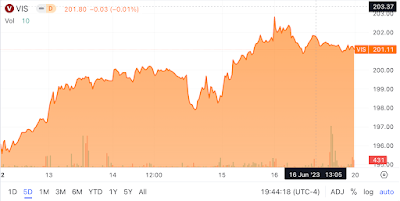Showing posts with label CMI. Show all posts
Showing posts with label CMI. Show all posts

## Saturday, November 26, 2022

### Beta of Cummins Included in the List

Here's the latest list of beta values. I have included Cummins on this list, and the returns for Cummins and the Vanguard S&P 500 Index are based on November 25, 2022, closing prices. Click on the image to see an enlarged version.

Exhibit: Beta Values for Cummins Included in the list

Companies in this list:

• Cisco Systems
• Colgate-Palmolive
• Lennox International
• Sealed Air
• Boeing
• Newell Brands
• Timken
• Cummins

### Monthly Return Comparison Between Cummins and Vanguard S&P 500 Index ETF

The following chart shows the Vanguard S&P 500 Index ETF (VOO) monthly returns on the x-axis and Cummins (CMI) on the y-axis. The regression line on the graph shows a steep slope, and the Pearson correlation value is 0.7. This value shows a very strong correlation between the monthly returns of the Vanguard S&P 500 Index ETF and Cummins. The p-value is significant at a 95% confidence interval. Cummins has returned 13.9% in the past year, while the Vanguard S&P 500 Index ETF has returned a -12.4%.

Exhibit: Monthly Returns of Vanguard S&P 500 Index ETF and Cummins [June 2019 - October 2022]

> # Create a new Graph of \$VOO and \$CMI Monthly Returns as Percentages
> ggscatter(df1, x = 'VOO_Monthly_Return', y = 'CMI_Monthly_Return',
+           add = "reg.line", conf.int = TRUE,
+           cor.coef = TRUE, cor.method = "pearson",
+           xlab = "VOO ETF Monthly Returns (%)", ylab = "CMI Monthly +           Returns (%)")

A linear regression of the monthly returns of the Vanguard S&P 500 Index ETF and Cummins shows the beta (coefficient of Vanguard S&P 500 Index ETF) for Cummins' monthly returns compared to the Vanguard ETF.

# Conduct the Linear Regression of the Monthly Returns Between \$VOO and \$CMI
lmVOOCMI = lm(CMI_Monthly_Return~VOO_Monthly_Return, data = VOOandCMI)
# Present the summary of the results from the linear regression
summary(lmVOOCMI)

Call:
lm(formula = CMI_Monthly_Return ~ VOO_Monthly_Return, data = VOOandCMI)

Residuals:
Min        1Q    Median        3Q       Max
-0.123268 -0.051037  0.004537  0.047062  0.115727

Coefficients:
Estimate Std. Error t value Pr(>|t|)
(Intercept)        0.005104   0.009226   0.553    0.583
VOO_Monthly_Return 0.993327   0.160541   6.187 2.84e-07 ***
---
Signif. codes:  0 ‘***’ 0.001 ‘**’ 0.01 ‘*’ 0.05 ‘.’ 0.1 ‘ ’ 1

Residual standard error: 0.05819 on 39 degrees of freedom
Multiple R-squared:  0.4954, Adjusted R-squared:  0.4824
F-statistic: 38.28 on 1 and 39 DF,  p-value: 2.845e-07

Cummins has a beta of 0.99 (nearly 1). Given this beta, Cummins, on average, moves in line with the S&P 500 Index. The adjusted R-squared value of 0.48 shows that about 48% of Cummins' monthly returns can be attributed to the returns of the S&P 500 Index. The p-value of 2.84e-07 shows that the regression analysis results are significant at the 95% confidence interval.

The average monthly return for Cummins between June 2019 and October 2022 was 1.49%. A one-sample t-test shows that the monthly return falls between -1.05% and 4.04%. But, the p-value of 0.99 is much higher than 0.05. This t-test may not be significant in the 95% confidence interval.

#
# One Sample t-test of \$CMI average monthly returns.
# Step 1:
# Copy Dataframe Column CMI_Monthly_Return into a List
#
CMI_Monthly_Return_Col <- c(VOOandCMI['CMI_Monthly_Return'])
# CMI_Monthly_Return_Col is a list object, but needs to be numeric
# for qqnorm to work.
#
typeof(CMI_Monthly_Return_Col)
# Convert List Object into a Column of doubles as as.numeric and unlist
#
y_CMI_Monthly_Return_Col <- as.numeric(unlist(CMI_Monthly_Return_Col))
typeof(y_CMI_Monthly_Return_Col)
# Let’s check if the data comes from a normal distribution
# using a normal quantile-quantile plot.
# Source: https://cran.r-project.org/web/packages/distributions3/vignettes/one-sample-t-test.html

Exhibit: Check if Cummins' Monthly Returns are Normally Distributed before doing a t-test

#
qqnorm(y_CMI_Monthly_Return_Col)
qqline(y_CMI_Monthly_Return_Col)
# Conduct a t-test to see if the population mean is 1.49% [0.0149]
#
t.test(y_CMI_Monthly_Return_Col, mu = .0149)

One Sample t-test

data:  y_CMI_Monthly_Return_Col
t = 0.0045069, df = 40, p-value = 0.9964
alternative hypothesis: true mean is not equal to 0.0149
95 percent confidence interval:
-0.01057188  0.04048574
sample estimates:
mean of x
0.01495693

### The Industrials Sector is on a Tear

The Vanguard Industrials Index ETF ( VIS ) touched a 52-week high of \$202.86 on Friday, June 16 (Exhibit 1) .   Exhibit 1: Vanguard Industr...